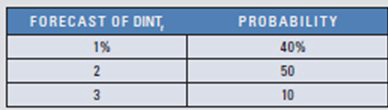# Forward versus Option Hedge As treasurer of Tempe Corp., you are confronted with the following problem. Assume the one-year forward rate of the British pound is \$1.59. You plan to receive 1 million pounds in one year. A one-year put option is available; it has an exercise price of \$1.61. The spot rate as of today is \$1.62, and the option premium is \$0.04 per unit. Your forecast of the percentage change in the spot rate was determined from the following regression model: e t = a 0 + a 1 D I N F t − 1 + a 2 D I N T t + μ where: e t = percentage change in the value of the British pound over period t DINF t − 1 = differential in inflation between the United States and the United Kingdom in period t − 1 DINT t = average differential between the U.S.interest rate and the British interest rate over period t a 0 , a 1 , and a 2 = regression coefficients μ = error term The regression model was applied to historical annual data, and the regression coefficients were estimated as follows: a 0 = 0.0 a 1 = 1.1 a 2 = 0.6 Assume last year’s inflation rates were 3 percent for the United States and 8 percent for the United Kingdom. Also assume that the interest rate differential(DINT) is forecasted as follows for this year: Using any of the available information, should you as treasurer choose the forward hedge or the put option hedge? Show your work.FindFind

### International Financial Management

14th Edition
Madura
Publisher: Cengage
ISBN: 9780357130698FindFind

### International Financial Management

14th Edition
Madura
Publisher: Cengage
ISBN: 9780357130698

#### Solutions

Chapter 11, Problem 35QA
Textbook Problem

## Forward versus Option Hedge As treasurer of Tempe Corp., you are confronted with the following problem. Assume the one-year forward rate of the British pound is \$1.59. You plan to receive 1 million pounds in one year. A one-year put option is available; it has an exercise price of \$1.61. The spot rate as of today is \$1.62, and the option premium is \$0.04 per unit. Your forecast of the percentage change in the spot rate was determined from the following regression model: e t = a 0 + a 1 D I N F t − 1 + a 2 D I N T t + μ where: e t = percentage change in the value of the British pound over period t DINF t − 1 = differential in inflation between the United States and the UnitedKingdom in period t − 1 DINT t = average differential between the U.S.interest rate and the British interest rate over period t a 0 , a 1 , and a 2 = regression coefficients μ = error termThe regression model was applied to historical annual data, and the regression coefficients were estimated as follows: a 0 = 0.0 a 1 = 1.1 a 2 = 0.6 Assume last year’s inflation rates were 3 percent for the United States and 8 percent for the United Kingdom. Also assume that the interest rate differential(DINT) is forecasted as follows for this year:Using any of the available information, should you as treasurer choose the forward hedge or the put option hedge? Show your work.

This textbook solution is under construction.

## Expert Solution

### Want to see the full answer?

Check out a sample textbook solution.

### Want to see this answer and more?

Experts are waiting 24/7 to provide step-by-step solutions in as fast as 30 minutes!*

*Response times vary by subject and question complexity. Median response time is 34 minutes and may be longer for new subjects.© 2021 bartleby. All Rights Reserved.